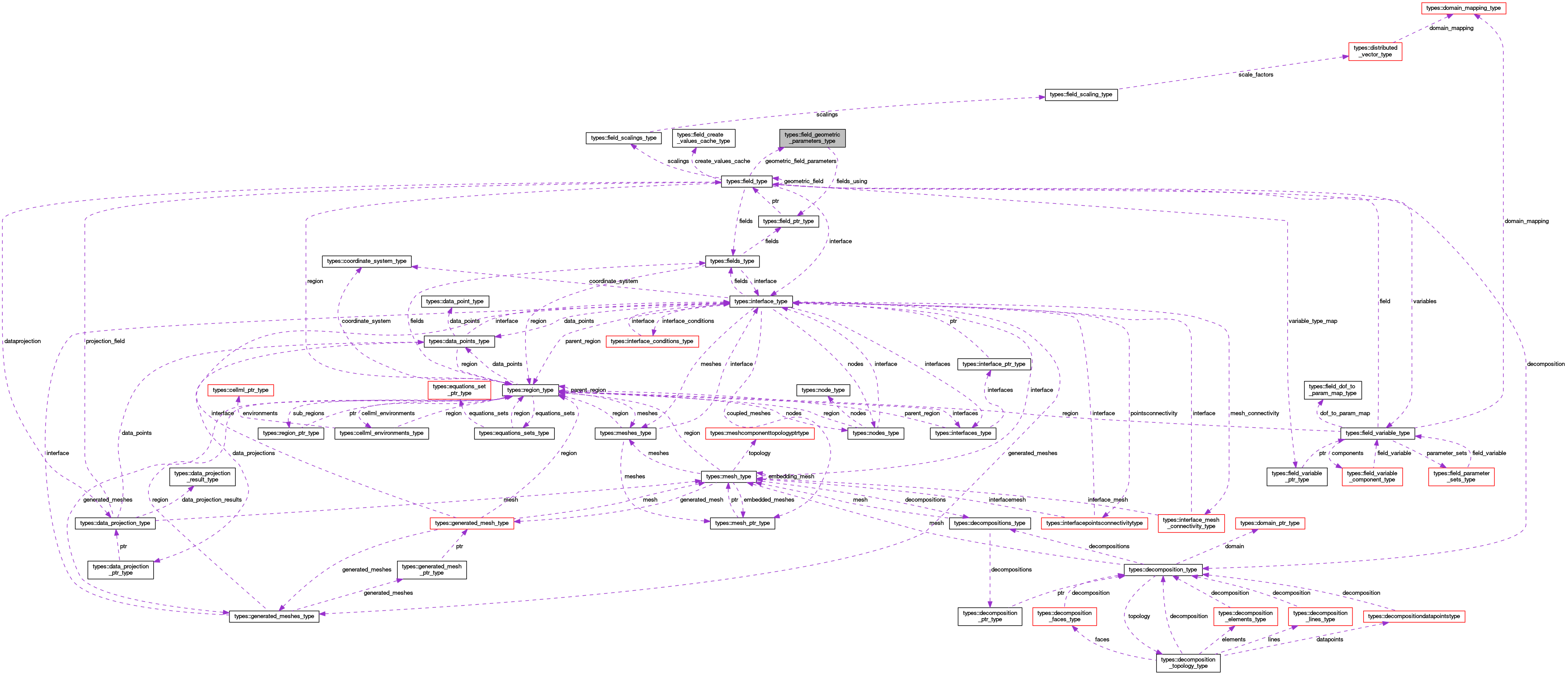OpenCMISS-Iron Internal API Documentation
types::field_geometric_parameters_type Type Reference

Contains the geometric parameters (lines, faces, volumes etc.) for a geometric field decomposition. More...

Collaboration diagram for types::field_geometric_parameters_type:[legend]

## Public Attributes

integer(intg) number_of_lines
The number of lines in the field. More...

integer(intg) number_of_areas
The number of areas in the field. More...

integer(intg) number_of_volumes
The number of volumes in the field. Inherited from the field decomposition. More...

real(dp), dimension(:), allocatable lengths
LENGTHS(nl). The length of the nl'th line in the field decomposition. More...

real(dp), dimension(:), allocatable areas
AREAS(nf). The area of the nf'th face in the field decomposition. More...

real(dp), dimension(:), allocatable volumes
VOLUMES(ne). The volume of the ne'th element in the field decomposition. More...

integer(intg) number_of_fields_using
The number of fields that use these geometric parameters for their scaling. More...

type(field_ptr_type), dimension(:), pointer fields_using
FIELDS_USINGS(field_idx). A pointer to the field_idx'th field that uses these geometric parameters for its scaling. More...

## Detailed Description

Contains the geometric parameters (lines, faces, volumes etc.) for a geometric field decomposition.

Definition at line 1156 of file types.f90.

## Member Data Documentation

 real(dp), dimension(:), allocatable types::field_geometric_parameters_type::areas

AREAS(nf). The area of the nf'th face in the field decomposition.

Definition at line 1161 of file types.f90.

 type(field_ptr_type), dimension(:), pointer types::field_geometric_parameters_type::fields_using

FIELDS_USINGS(field_idx). A pointer to the field_idx'th field that uses these geometric parameters for its scaling.

Definition at line 1164 of file types.f90.

 real(dp), dimension(:), allocatable types::field_geometric_parameters_type::lengths

LENGTHS(nl). The length of the nl'th line in the field decomposition.

Definition at line 1160 of file types.f90.

 integer(intg) types::field_geometric_parameters_type::number_of_areas

The number of areas in the field.

Definition at line 1158 of file types.f90.

 integer(intg) types::field_geometric_parameters_type::number_of_fields_using

The number of fields that use these geometric parameters for their scaling.

Definition at line 1163 of file types.f90.

 integer(intg) types::field_geometric_parameters_type::number_of_lines

The number of lines in the field.

Definition at line 1157 of file types.f90.

 integer(intg) types::field_geometric_parameters_type::number_of_volumes

The number of volumes in the field. Inherited from the field decomposition.

Definition at line 1159 of file types.f90.

 real(dp), dimension(:), allocatable types::field_geometric_parameters_type::volumes

VOLUMES(ne). The volume of the ne'th element in the field decomposition.

Definition at line 1162 of file types.f90.